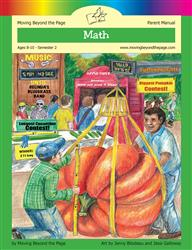Age 5-7 Reading Is Now Available!

# Common Core Alignment

CCSS.Math.Content.4.NBT.2 - Read and write multi-digit whole numbers using base-ten numerals, number names, and expanded form. Compare two multi-digit numbers based on meanings of the digits in each place, using >, =, and < symbols to record the results of comparisons.

## 5: MathUnit 1: Place Value to 1,000,000
Lesson 1: Numbers to 10,000 Review
Lesson 10: Unit Test
Lesson 3: The Thousands Places
Lesson 4: One Million
Lesson 5: Comparing Big Numbers
Lesson 6: Rounding Big Numbers
Final Project: Cross Number PuzzleUnit 9: Skills Review
Lesson 1: Multi-Digit Multiplication and Division